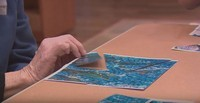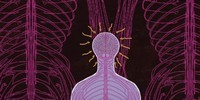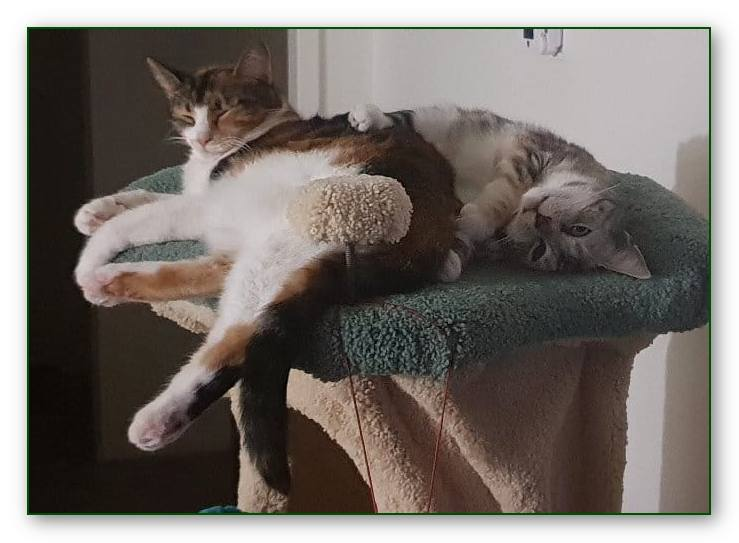Like   Tweet   Pin   +1   in
 /* styles */ Stories, Tips and Resources   ||   Issue #13 March 2019It's been a while since the last issue of Good Chi News. But I'm back on track!

This issue includes the usual mix of resources, reflections and tidbits - as well as the latest news regarding our programs for 2019.

Regards
Chris Bennett
Chris Chi

 table div table+table+table+table div table{width:100%;padding:0}table div table+table+table+table div table img{width:96.23%;padding:0;float:none}table div table+table+table+table div table td{width:100%;padding:0 1.88% 18px}/* styles */## An "AHA" ExperienceEven though I've run over 1200 chair chi workshops in six years in the aged care sector I'm still experiencing 'aha' moments when teaching Chair Chi to residents at all levels of care.

That ‘aha’ moment has given me another technique to use for residents who have hearing problems, as well as for those who are not cognitively aware enough to follow verbal instructions. Read more ...

 table div table+table+table+table+table+table+table div table{width:100%;padding:0}table div table+table+table+table+table+table+table div table img{width:96.23%;padding:0;float:none}table div table+table+table+table+table+table+table div table td{width:100%;padding:0 1.88% 18px}/* styles */## What's NewBoth our complete Chair Chi Training Program and our Ease Pain the Chi Way workshop have been reviewed, revised and enhanced in 2019.

Our focus for this year will be on delivering onsite workshops for aged care organisations. which is a cost effective and convenient way of learning as compared to sending individuals to a public workshop.

Here's the latest information about our workshops for 2019 and an upate for previous participants.

 table div table+table+table+table+table+table+table+table+table+table div table{width:100%;padding:0}table div table+table+table+table+table+table+table+table+table+table div table img{width:96.23%;padding:0;float:none}table div table+table+table+table+table+table+table+table+table+table div table td{width:100%;padding:0 1.88% 18px}/* styles */## Chair Chi Plus Pilot Study Report

 /* styles */ The Chair Chi Plus Pilot Study was conducted at a local aged care facility here in Melbourne. It was developed with the purpose of improving residents' overall well-being: physically - psychologically, emotionally and socially. Residents who participated in the study were aged from 82 to 93, all with high care needs.
 table div table+table+table+table+table+table+table+table+table+table+table+table+table div table{width:100%;padding:0}table div table+table+table+table+table+table+table+table+table+table+table+table+table div table img{width:96.23%;padding:0;float:none}table div table+table+table+table+table+table+table+table+table+table+table+table+table div table td{width:100%;padding:0 1.88% 18px}/* styles */## Mindfulness: Improving Sports Performance & Reducing Sport AnxietyIn this article Crystal Chariton explores mindfulness as it relates to sport performance, including:

(a) dispositional mindfulness;
(b) mindfulness-based interventions; and
(c) sport anxiety.

There are themes in this article that definitely resonate with me as a former basketball coach and current Tai Chi teacher!

 table div table+table+table+table+table+table+table+table+table+table+table+table+table+table+table+table div table{width:100%;padding:0}table div table+table+table+table+table+table+table+table+table+table+table+table+table+table+table+table div table img{width:96.23%;padding:0;float:none}table div table+table+table+table+table+table+table+table+table+table+table+table+table+table+table+table div table td{width:100%;padding:0 1.88% 18px}/* styles */## Dementia: Filling the Day With MeaningHere is an excellent short clip, very useful for anyone in the Aged Care sector, in which Teepa Snow discusses and provides examples of how a person with dementia in an aged care facility can 'fill the day with meaning'. Read More ...

 table div table+table+table+table+table+table+table+table+table+table+table+table+table+table+table+table+table+table+table div table{width:100%;padding:0}table div table+table+table+table+table+table+table+table+table+table+table+table+table+table+table+table+table+table+table div table img{width:96.23%;padding:0;float:none}table div table+table+table+table+table+table+table+table+table+table+table+table+table+table+table+table+table+table+table div table td{width:100%;padding:0 1.88% 18px}/* styles */## Brain PracticeOne way to help improve your performance when practicing physical skills such as sport or music etc. is to visualise the skills taught. In other words "practice in your brain".

It's something I do on a regular basis when I'm practicing various aspects of Tai Chi.

Annie Bosler and Don Greene have produced a very good short TED Ed clip on 'How To Practice Effectively ... for just about anything'.

 table div table+table+table+table+table+table+table+table+table+table+table+table+table+table+table+table+table+table+table+table+table+table div table{width:100%;padding:0}table div table+table+table+table+table+table+table+table+table+table+table+table+table+table+table+table+table+table+table+table+table+table div table img{width:96.23%;padding:0;float:none}table div table+table+table+table+table+table+table+table+table+table+table+table+table+table+table+table+table+table+table+table+table+table div table td{width:100%;padding:0 1.88% 18px}/* styles *//* styles */ “Let it be still, and it will gradually become clear.” ( Lao Tzu, from Tao Te Ching)
 table div table+table+table+table+table+table+table+table+table+table+table+table+table+table+table+table+table+table+table+table+table+table+table+table div table{width:100%;padding:0}table div table+table+table+table+table+table+table+table+table+table+table+table+table+table+table+table+table+table+table+table+table+table+table+table div table img{width:96.23%;padding:0;float:none}table div table+table+table+table+table+table+table+table+table+table+table+table+table+table+table+table+table+table+table+table+table+table+table+table div table td{width:100%;padding:0 1.88% 18px}/* styles */## Cat ChiTabitha & Tully demonstrating (Cat) Chi.

Total relaxation!

Something that cats do so well and we can benefit from learning. :)

 table div table+table+table+table+table+table+table+table+table+table+table+table+table+table+table+table+table+table+table+table+table+table+table+table+table+table+table div table{width:100%;padding:0}table div table+table+table+table+table+table+table+table+table+table+table+table+table+table+table+table+table+table+table+table+table+table+table+table+table+table+table div table img{width:96.23%;padding:0;float:none}table div table+table+table+table+table+table+table+table+table+table+table+table+table+table+table+table+table+table+table+table+table+table+table+table+table+table+table div table td{width:100%;padding:0 1.88% 18px}/* styles *//* styles */